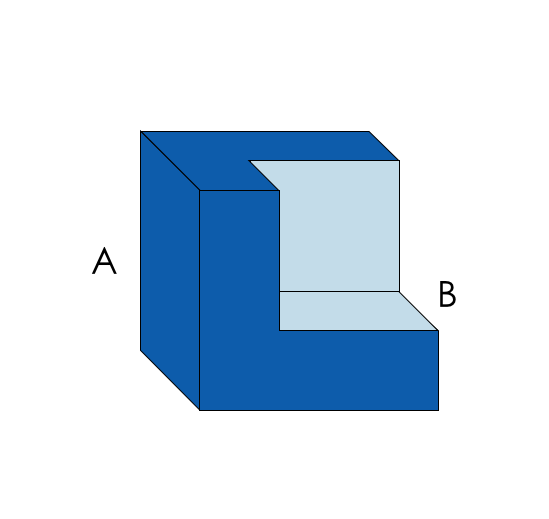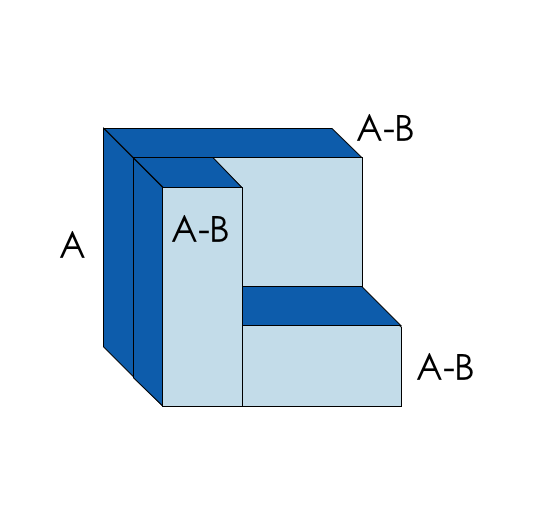# Polynomials

## Objective

Factor difference and sums of cubes. Explain sums and differences of cubes as polynomial identities.

## Common Core Standards

### Core Standards

?

• A.APR.C.4 — Prove polynomial identities and use them to describe numerical relationships. For example, the polynomial identity (x² + y²)2 = (x² — y²)² + (2xy)² can be used to generate Pythagorean triples.

?

• A.SSE.A.2

## Criteria for Success

?

1. Explain that there are factoring patterns that are referred to as “identities” and that these identities are true regardless of the value of $x$ or $y$ in the polynomial function.
2. Describe how the polynomial identities can make factoring easier and can be proven through already known algebraic methods.
3. Use the polynomial identities of sums and differences of cubes to solve problems.
4. Derive the difference of cubes identity as ${(a-b)(a^2-ab+b^2 )}$
5. Derive the sum of cubes identity as ${(a+b)(a^2+ab+b^2 )}$.

## Tips for Teachers

?

• Memorization of the difference and sums of cubes identity is not necessary to master this lesson.
• This resource describes how long division can be used to solve Anchor Problem #1

## Anchor Problems

?

### Problem 1

The diagram below shows a cube of dimensions $B$ subtracted from a cube of dimensions $A$. The total volume represents $A^3-B^3$The diagram below shows the volume of $A^3-B^3$ subdivided into smaller volumes. Find the formula for the difference of two cubes using the smaller volumes shown.#### References

YouTube Video: “Difference of Cubes” by Alex Toole

### Problem 2

Determine if the following are factors of the cubic polynomials shown:

Is ${(a-b)}$ a factor of ${a^3-b^3}$

Is ${(a+b)}$ a factor of ${a^3+b^3}$

## Problem Set

?

The following resources include problems and activities aligned to the objective of the lesson that can be used to create your own problem set.

• Include problems where students need to factor the difference or sum of two cubes with and without leading coefficients.

${16x^6+2y^3}$
${16x^6-2y^3}$